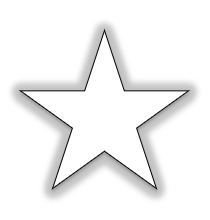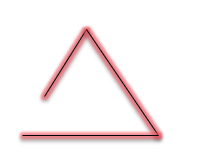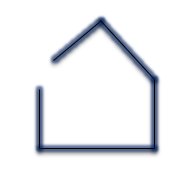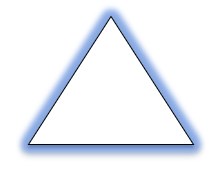# Open and Closed Figures | Examples | Maths

Open and Closed Figures

#### Open Figure

If starting point and the end point of a figure are not same, then the figure is an Open Figure.

#### Closed Figure

If starting point and the end point of a figure are same, then the figure is a Closed Figure.

#### Open and Closed Figures – Examples

Example 1

Is the given figure a closed figure?Explanation:

If starting point and the end point of a figure are same, then the figure is a closed figure.In the given figure, starting and end point of a figure are same.

Hence, the given figure is a closed figure.

Example 2

Is the given figure is an open figure?Explanation:

If starting point and the end point of a figure are not same, then the figure is an open figure.In the given figure, starting and end point of a figure are not same.

Hence, the given figure is an open figure.

Example 3

Is the given figure a closed figure?Explanation:

If starting point and the end point of a figure are same, then the figure is a closed figure.In the given figure, starting and end point of a figure are not same.

Hence, the given figure is not a closed figure.

Example 4

Is the given figure is an open figure?Explanation:

If starting point and the end point of a figure are not same, then the figure is an open figure.In the given figure, starting and end point of a figure are same.

Hence, the given figure is not an open figure.